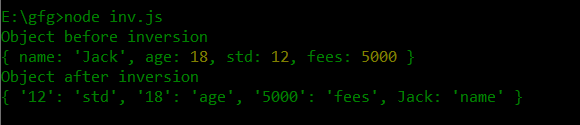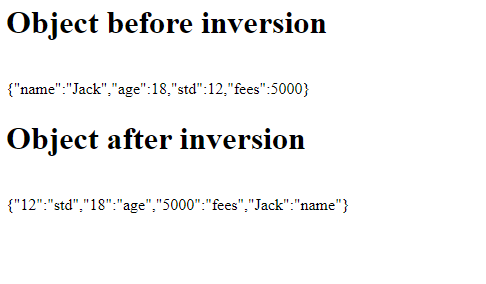# How to invert key value in JavaScript object ?

• Last Updated : 03 Dec, 2020

JavaScript is a high level, interpreted and dynamically typed client-side scripting language. JavaScript is used to add dynamic features to the static HTML. Everything is an object in JavaScript. Objects in JavaScript can be declared using figure brackets {..} and the objects may comprise certain properties. These properties are basically key-value pairs. The key is an identifier which is used to store and retrieve values. Inverting key-value pairs is tedious using conventional methods. But with the advent of “underscore.js”, inversion of key values can be performed using the inbuilt method _.invert(). In this article, we shall discuss both the methods of inverting key value pairs of JavaScript objects.

First Approach: In this example, we will demonstrate the conventional method of inverting key-value pairs. At first, a student object is created with properties “name”, “age”, “std” and “fees”. An inverse() function is defined which takes the student object as a parameter and loops through each key of the object. A new object retobj is defined which stores the inverted key value pairs.

Code Implementation:

## Javascript

 `function` `inverse(obj){ ` `  ``var` `retobj = {}; ` `  ``for``(``var` `key ``in` `obj){ ` `    ``retobj[obj[key]] = key; ` `  ``} ` `  ``return` `retobj; ` `} ` ` `  `var` `student =  ` `{ ` `    ``name : ``"Jack"``, ` `    ``age: 18, ` `    ``std : 12, ` `    ``fees : 5000 ` `} ` `console.log(``"Object before inversion"``); ` `console.log(student); ` `student = inverse(student); ` `console.log(``"Object after inversion"``); ` `console.log(student); `

Output:Second Approach: In this example, we use the _.invert() method of “underscore.js” to invert the key value pairs of the object. The method takes the object as a parameter and returns a copy of the object with the keys as values and values as keys. The “student” object is passed to the _.invert() method. The method returns the inverted copy of the “student” object. The program imports the external “underscore.js” library to use inbuilt methods. The results are displayed on the webpage.

Syntax:

`_.invert(object)`

Code Implementation:

## HTML

 ` ` `<``html``> ` ` `  `<``head``>  ` `    ``<``script` `        ``src``= ` `"https://cdn.jsdelivr.net/npm/underscore@1.12.0/underscore-min.js"``> ` `    `` ` ` ` ` `  `<``body``> ` `    ``<``script` `type``=``"text/javascript"``> ` `        ``var student = { ` `            ``name : "Jack", ` `            ``age: 18, ` `            ``std : 12, ` `            ``fees : 5000 ` `        ``} ` ` `  `        ``document.write("<``h1``>Object before inversion"); ` `        ``document.write("<``br``>"); ` `        ``document.write(JSON.stringify(student)); ` `        ``document.write("<``br``>"); ` `        ``student = JSON.stringify(_.invert(student)); ` `        ``document.write("<``h1``>Object after inversion"); ` `        ``document.write("<``br``>"); ` `        ``document.write(student); ` `    `` ` ` ` ` `  ` `

Output:My Personal Notes arrow_drop_up
Recommended Articles
Page :